• Save

# Verbal Reasoning Classification Questions and Answers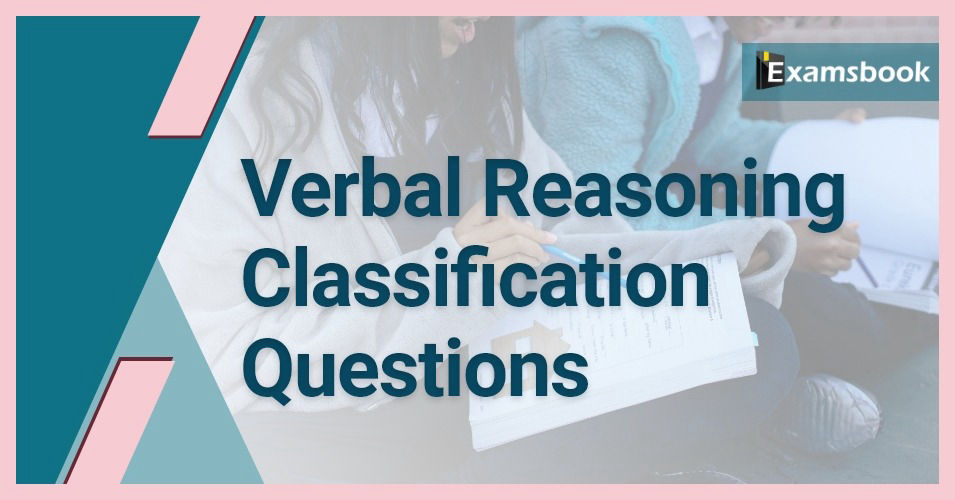Classification Problems are important from a competitive exam point of view. Here I am providing Classification Questions and answers to solve. Practice with selective Classification Questions for competitive exams. Verbal Reasoning Classification Questions and Answers for all Exams like CAT,MAT,XAT,GRE,GMAT,MBA,MCA,Bank Exams,Bank PO,SBI,Gate,Nda,Ssc. Classification questions are all frequently asked in competitive Exams.

Instead of that, you can read or practice of all the topics of reasoning by visit on Reasoning Questions and Answers for bank exams.

## Classification Problems: Verbal Reasoning Classification Questions and Answers

Q :

Find the odd image in the following diagram?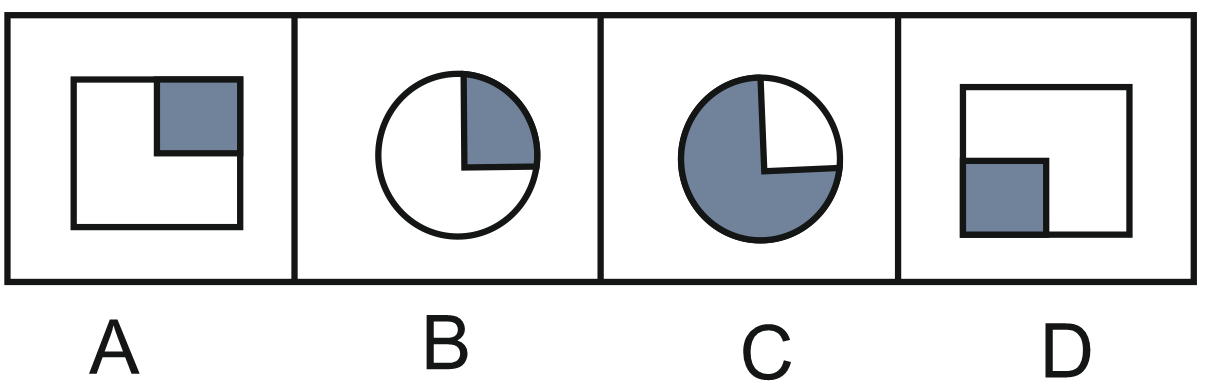(A) A

(B) C

(C) B

(D) D

Q :

Find out the odd word/letters/ number/number pair from the given alternatives.

(C) Dhyan Chand

(D) Yuvraj Singh

Q :

In following questions select the one which is different from other three responses.

(A) I

(B) E

(C) B

(D) O

Q :

In each of the following select the one which is different from other three responses.

(A) 88

(B) 97

(C) 132

(D) 121

Q :

Select the odd word/ letters/ number/ number pair from the given alternative.
(A) 1728
(B) 4096
(C) 5832
(D) 2746

(A) A

(B) B

(C) C

(D) D

Q :

Find the odd word from the following figure?

(A)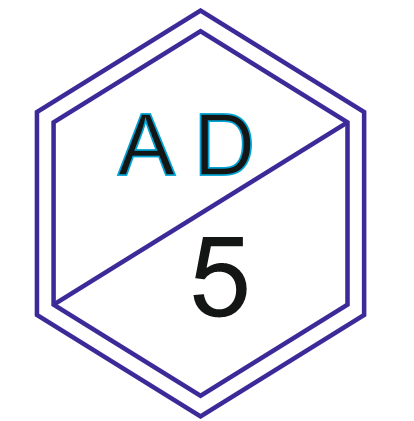(B)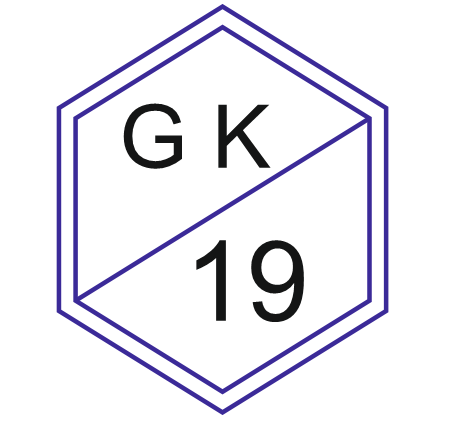(C)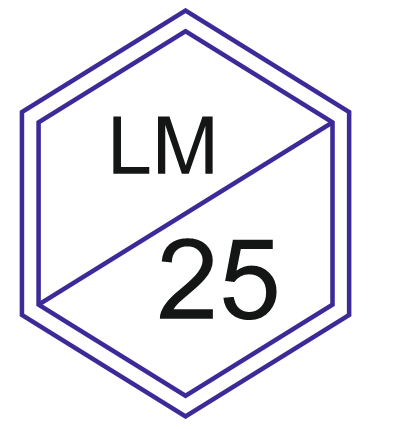(D)Q :

Select the odd word/letters/number/number pair from the given alternatives.

(A) Perpendicular

(B) Base

(C) Hypotenuse